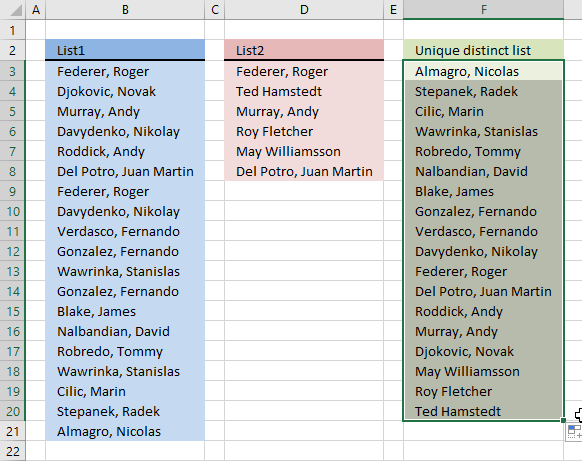Author: Oscar Cronquist Article last updated on December 29, 2018Question: I have two ranges or lists (List1 and List2) from where I would like to extract a unique distinct list? Merge two lists without duplicates, in other words.

Update! 2017-09-01, smaller regular formula in cell F3:

=IFERROR(LOOKUP(2, 1/(COUNTIF(\$F\$2:F2,\$B\$3:\$B\$21)=0), \$B\$3:\$B\$21), LOOKUP(2, 1/(COUNTIF(\$F\$2:F2, \$D\$3:\$D\$8)=0), \$D\$3:\$D\$8))

### Explaining regular formula in cell F3

This formula consists of two similar parts, one returns values from List1 and the other returns values from List2.

#### Step 1 - Prevent duplicate values

The COUNTIF function counts values based on a condition, in this case, I am counting values in cells above. This makes sure that duplicates are ignored.

COUNTIF(\$F\$2:F2,\$B\$3:\$B\$21)=0

becomes

COUNTIF("Unique distinct list",{"Federer, Roger ";"Djokovic, Novak ";"Murray, Andy ";"Davydenko, Nikolay ";"Roddick, Andy ";"Del Potro, Juan Martin ";"Federer, Roger ";"Davydenko, Nikolay ";"Verdasco, Fernando ";"Gonzalez, Fernando ";"Wawrinka, Stanislas ";"Gonzalez, Fernando ";"Blake, James ";"Nalbandian, David ";"Robredo, Tommy ";"Wawrinka, Stanislas ";"Cilic, Marin ";"Stepanek, Radek ";"Almagro, Nicolas "})=0

becomes

COUNTIF("Unique distinct list",{"Federer, Roger "; "Djokovic, Novak ";"Murray, Andy ";"Davydenko, Nikolay "; "Roddick, Andy ";"Del Potro, Juan Martin "; "Federer, Roger ";"Davydenko, Nikolay ";"Verdasco, Fernando ";"Gonzalez, Fernando ";"Wawrinka, Stanislas ";"Gonzalez, Fernando "; "Blake, James ";"Nalbandian, David ";"Robredo, Tommy "; "Wawrinka, Stanislas ";"Cilic, Marin ";"Stepanek, Radek ";"Almagro, Nicolas "})=0

{0;0;0;0;0;0;0;0;0;0;0;0;0;0;0;0;0;0;0}=0

and returns

{TRUE;TRUE;TRUE; TRUE;TRUE;TRUE;TRUE; TRUE;TRUE;TRUE;TRUE; TRUE;TRUE;TRUE;TRUE; TRUE;TRUE;TRUE;TRUE}

#### Step 2 - Divide 1 with array

The LOOKUP function ignores error and if we divide 1 with 0 an error occurs. 1/0 = #DIV/0!

1/(COUNTIF(\$F\$2:F2,\$B\$3:\$B\$21)=0)

becomes

1/{TRUE;TRUE;TRUE; TRUE;TRUE;TRUE;TRUE; TRUE;TRUE;TRUE;TRUE; TRUE;TRUE;TRUE;TRUE; TRUE;TRUE;TRUE;TRUE}

and returns

{1;1;1;1; 1;1;1;1;1; 1;1;1;1;1;1; 1;1;1;1}

#### Step 3 - Return value based on array

LOOKUP(2, 1/(COUNTIF(\$F\$2:F2,\$B\$3:\$B\$21)=0), \$B\$3:\$B\$21)

becomes

LOOKUP(2, {1;1;1;1; 1;1;1;1;1; 1;1;1;1;1;1; 1;1;1;1}, \$B\$3:\$B\$21)

becomes

LOOKUP(2, {1;1;1;1; 1;1;1;1;1; 1;1;1;1;1;1; 1;1;1;1}, {"Federer, Roger ";"Djokovic, Novak ";"Murray, Andy ";"Davydenko, Nikolay ";"Roddick, Andy ";"Del Potro, Juan Martin ";"Federer, Roger ";"Davydenko, Nikolay ";"Verdasco, Fernando ";"Gonzalez, Fernando ";"Wawrinka, Stanislas ";"Gonzalez, Fernando ";"Blake, James ";"Nalbandian, David ";"Robredo, Tommy ";"Wawrinka, Stanislas ";"Cilic, Marin ";"Stepanek, Radek ";"Almagro, Nicolas "})

and returns Almagro, Nicolas in cell F3.

#### Step 4 - Return values from List2

When values run out from List1 formula1 returns errors, the IFERROR function then moves to formula2.

IFERROR(formula1, formula2)

formula2 is just like formula1 except that it returns values from List2.

Array formula in F3:

=IFERROR(IFERROR(INDEX(\$B\$3:\$B\$21, MATCH(0, COUNTIF(\$C\$1:C1, \$B\$3:\$B\$21), 0)), INDEX(\$D\$3:\$D\$8, MATCH(0, COUNTIF(\$C\$1:C1, \$D\$3:\$D\$8), 0))), "")

How to create an array formula

1. Select cell C2
2. Click in formula bar3. Paste array formula to formula bar
4. Press and hold Ctrl+ Shift
5. Press Enter
6. Release all keys

Recommended article

How to enter an array formula

Array formulas allows you to do advanced calculations not possible with regular formulas.

How to copy formula

1. Copy cell C2
2. Select cell range C3:C19
3. Paste

Earlier versions of Excel 2007

Array formula in cell C2:

=IF(ISERROR(INDEX(\$A\$2:\$A\$20, MATCH(0, COUNTIF(\$C\$1:C1, \$A\$2:\$A\$20), 0))), INDEX(\$B\$2:\$B\$7, MATCH(0, COUNTIF(\$C\$1:C1, \$B\$2:\$B\$7), 0)), INDEX(\$A\$2:\$A\$20, MATCH(0, COUNTIF(\$C\$1:C1, \$A\$2:\$A\$20), 0)))